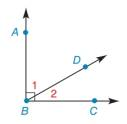Chapter 1.4, Problem 7E### Elementary Geometry for College St...

6th Edition
Daniel C. Alexander + 1 other
ISBN: 9781285195698

#### Solutions

Chapter
Section### Elementary Geometry for College St...

6th Edition
Daniel C. Alexander + 1 other
ISBN: 9781285195698
Textbook Problem
1 views

# In Exercises 5 to 8, describe in one word the relationship between the angles. ∠ 1 and ∠ 2To determine

To describe:

The relationship between the given angles.

Explanation

Given:

The given angles are,

1 and 2

The given figure is,

If the sum of two angles is 90°, then the angles are known as complementary angles

### Still sussing out bartleby?

Check out a sample textbook solution.

See a sample solution

#### The Solution to Your Study Problems

Bartleby provides explanations to thousands of textbook problems written by our experts, many with advanced degrees!

Get Started

#### Find more solutions based on key concepts